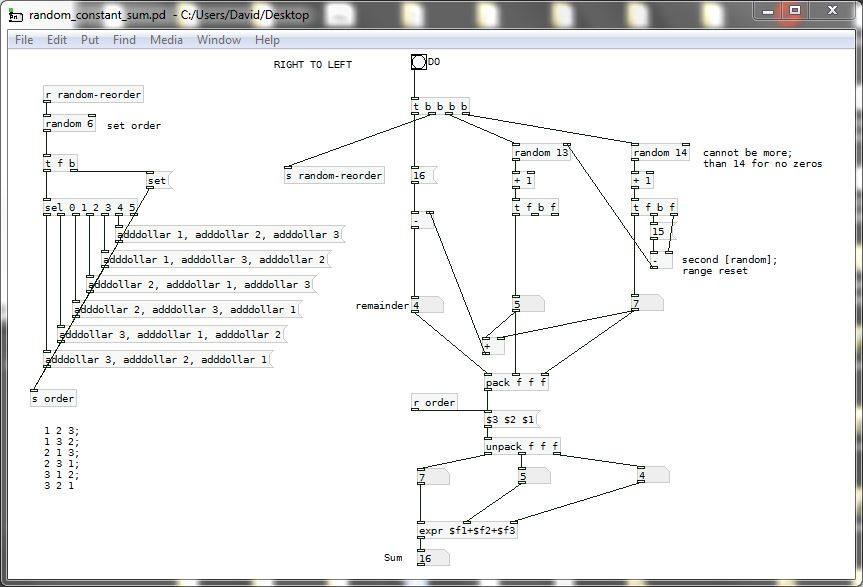• ### Random numbers that add up to a certain number

Hey guys, how can I randomly generate a number of values which add up to a given value? So say I want 4 numbers that add up to 16: they could be (4, 4, 4, 4) or (10, 2, 2, 2) or (4, 3, 5, 4) etc etc. does anyone know a way of doing this efficiently? I would also like to be able to control how many of these numbers are allowed to be zero, if any.

• Posts 11 | Views 1971
• so i implemented that like so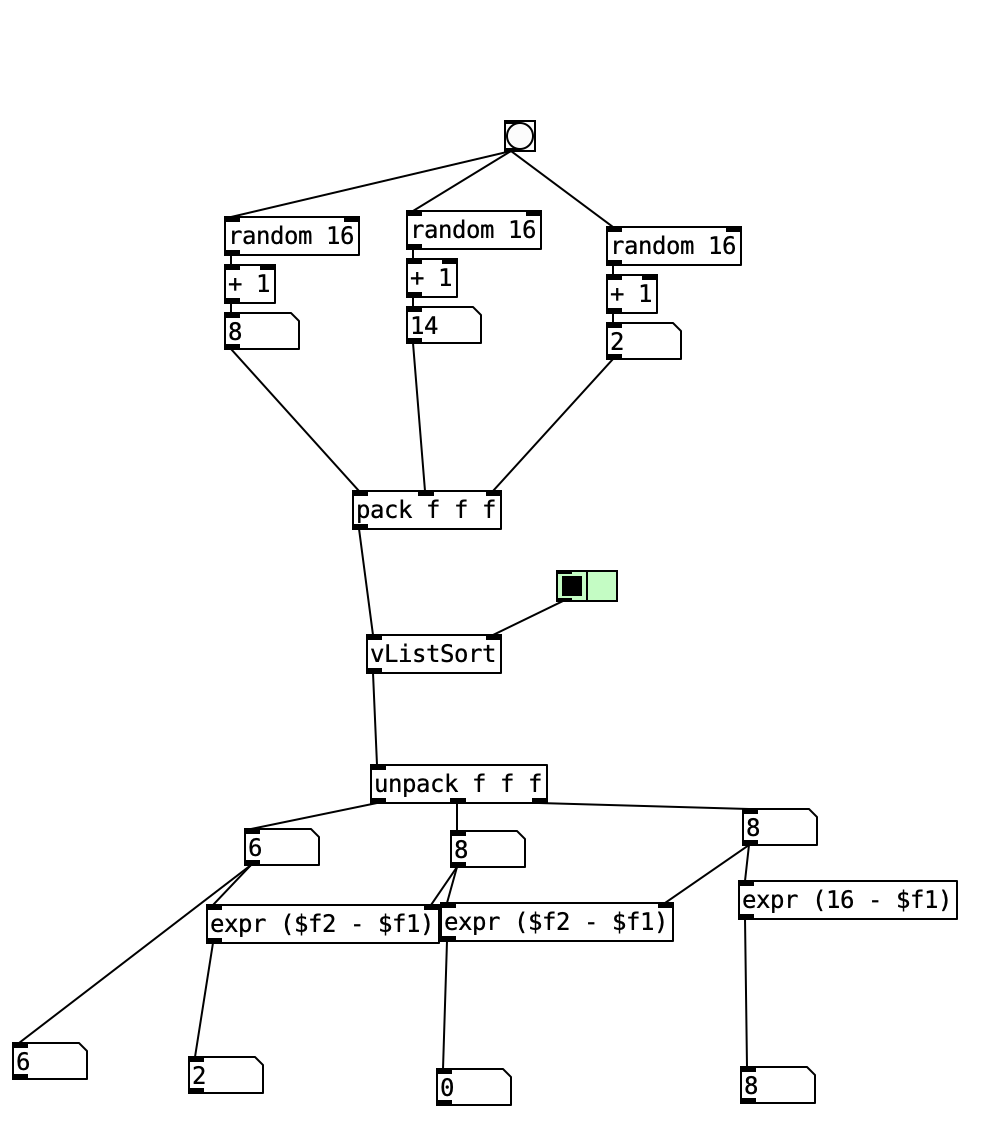just wondering if anyone knows how i could prevent zeros coming up? is there a way I could just re-run this code over and over if a zero comes up, without causing a stack overflow?

• Have you tried this approach < https://stackoverflow.com/a/8064754 >?

• @Nicolas-Danet
this is some good idea.... i was curious, so i just made a patch according that idea and somehow avoids zeros....however, the disadvantage is, that there are many repetitions in a list of output numbers.... maybe someone finds it useful....random_to_certain_sum.pd

• Here is another solution:
rand12.pd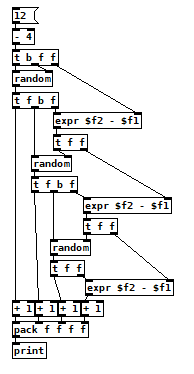• Here is another attempt (because the 9 is not possible in my first try... )
rand12b.pd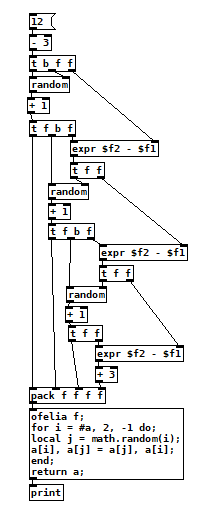There is also a fisher yates algorithm implemented with lua for randomizing the list.
The new problem is: the combinations of one 9 and three 1's seem over representated because every time there is a 9 there also need to be three 1's...
So perhaps my first attempt has a more balanced result.

The idea is to start with a list of zeros and add 1 randomly until the sum is reached.

Implementation: random_to_sum.pd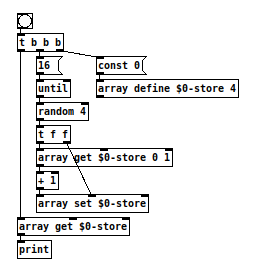• @ingox thats nice. i modified it a bit, to avoid zeros (but now the sum(16) needs to be at least as large as the list length(4) to give an accurate result):
random_to_sum_1.pd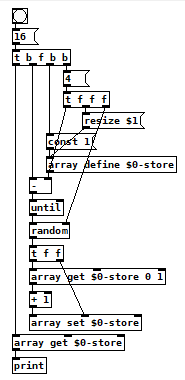• Without zeros: random_to_sum2.pd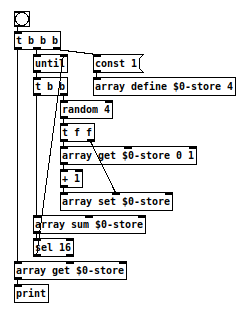Old school solution. I am sure the randomization re-ordering at the left has a more elegant solution and could be what [vListSort] does.
Only 3 numbers...... but the expansion methodology is obvious.......... except for the randomized list reordering which will be truly horrible with my method........ but see below....
Because the first number to drop cannot be greater than 14 (for "no zeroes") and then the second must be limited.......... random_constant_sum.pd
David.